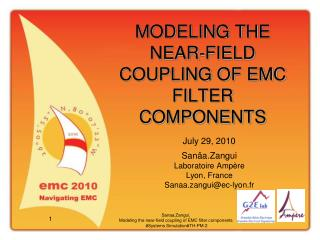DownloadDownload PresentationMODELING THE NEAR-FIELD COUPLING OF EMC FILTER COMPONENTS

# MODELING THE NEAR-FIELD COUPLING OF EMC FILTER COMPONENTS

Télécharger la présentation## MODELING THE NEAR-FIELD COUPLING OF EMC FILTER COMPONENTS

- - - - - - - - - - - - - - - - - - - - - - - - - - - E N D - - - - - - - - - - - - - - - - - - - - - - - - - - -
##### Presentation Transcript

1. MODELING THE NEAR-FIELD COUPLING OF EMC FILTER COMPONENTS July 29, 2010 Sanâa.Zangui LaboratoireAmpère Lyon, France Sanaa.zangui@ec-lyon.fr Sanaa.Zangui, Modeling the near-field coupling of EMC filter components #Systems Simulation#TH-PM-2

2. Outline • Introduction • Aims • Equivalent model • Validation • Summary • Questions Sanaa.Zangui, Modeling the near-field coupling of EMC filter components

3. Introduction • In Power electronic => noises • Lowpassfilter => reduceconducted noises(HF) by conduction Power electronic system Filter Motor by radiation Lowpassfilter Magnitude (dB) Frequency Sanaa.Zangui, Modeling the near-field coupling of EMC filter components

4. Introduction L DM • Magnetic Coupling Analysis (Low voltage/ high current) • Near-field Approximation. - Distance between components<< wavelenghtλ - Maximum frequency (100MHz) => λmax= 3meters • Parasitic parameters and EMC Filter Performances: - Self-parasitic - Filter components coupling. Cy Cy 1 2 EPC EPR L DM ESR ESR 2 1 M M 2 1 Cy Cy 2 1 ESL ESL 2 1 M 3 1 2 Magnitude (dB) Mutuals inductances The self-parasitics of components Frequency 30MHz 1MHz Sanaa.Zangui, Modeling the near-field coupling of EMC filter components

5. Aims Taking into account the effects of parasitic parameters in the first step of designer a filter • Equivalent Model Components : • Model the magnetic near-field produced by filter components • Take into account the near electromagnetic environment • Integrated in an electrical circuit software • Compute Coupling Effects • Using the equivalent model of components • According to their geometric placement. Sanaa.Zangui, Modeling the near-field coupling of EMC filter components

6. Equivalent model(Multipolar expansion) • 3D EM fields : multipolar expansion • The field is computed outside the sphere that contains the equivalent source. n=1 m=0 (dipole) n=2 m=0 (quadrupole) Sanaa.Zangui, Modeling the near-field coupling of EMC filter components

7. Equivalent model (Equivalent model) • The expression of the magnetic field : • Qnm are functions of H • Computing Hby using 3D numerical model or measurement Qnm are parameters which need to be identified => equivalent model of the radiated field component n: degree, m: azimuthal order, Ynm: The spherical harmonics functions Sanaa.Zangui, Modeling the near-field coupling of EMC filter components

8. Equivalent model (Mutual inductance) • Using the equivalent radiated field source model. • The spheres which contain each of the sources don’t intersect • The expression of the mutual inductance is: The coefficients of the multipolar expansion of sources 1 and 2 must be expressed in the same reference => translation. Sanaa.Zangui, Modeling the near-field coupling of EMC filter components

9. Equivalent model (Computing method) Component 1 Component 2 H1 field by numerical modeling or measurement H2field by numerical modeling or measurement The translation is based on the “Addition Theorem for Vector Spherical Harmonics” Q1nmComputation Q2nmComputation Equivalent model 1 Equivalent model 2 Rotation + translation in the reference1 of Q2nm=> Q’2nm Computation of the mutual inductance M in fonction of Q1nm and Q’2nm Sanaa.Zangui, Modeling the near-field coupling of EMC filter components

10. Equivalent model (Numerical modeling) • H field by FEM method(Flux3D®) To result this case : - Flux3D => 2000 unknows - Multipolar expansion : For n=Nmax=3 =>15unknows For n=Nmax=5 => 35unknows Infinite box Element to modeling Sphere of validity Normal component of H => Qnm=> equivalent model => mutual inductance Sanaa.Zangui, Modeling the near-field coupling of EMC filter components

11. Validation(case 1) • Our result was compared to the numerical result computed by FEM(Flux3D cedrat). • Two loops, C1 and C2 with a radius “Rspire” of 10 cm, separated by r • At r =0.2m the error is greater for Nmax=3 than Nmax=5 Relative error (%) Mutual inductance(H) Sanaa.Zangui, Modeling the near-field coupling of EMC filter components

12. Validation(case 2) • For the same previous loops C1 and C2 • For a rotation of 45° around the y axis of the loop C2 • The results are similar to the previous case Relative error (%) Mutual inductance(H) Sanaa.Zangui, Modeling the near-field coupling of EMC filter components

13. Summary • The method is validated : • Using multipolar expansion => equivalent model of the radiated field of components. • Using the equivalent model to compute the coupling (Mutual inductance) between components. • Apply this method to more complex components. • Measurement system that measures Qnmcomponents . • Couple this method with Partial Element Equivalent Circuit (PEEC)=> filter modeling including all coupling (track/track, components/components, components/track). • The cylindrical harmonics can be considered for modeling components such as capacitors or cables. • Provide component libraries including models of coupling between power electronic components. Sanaa.Zangui, Modeling the near-field coupling of EMC filter components

14. Questions Sanaa.Zangui, Modeling the near-field coupling of EMC filter components